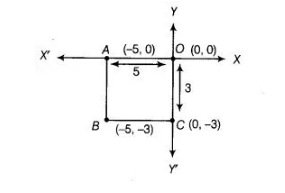# Write the coordinates of the vertices of`
Question:

Write the coordinates of the vertices of a rectangle whose length and breadth are 5 and 3 units respectively, one vertex at the origin, the longer side lies on the X-axis and one of the vertices lies in the third quadrant.Solution:

Given, length of a rectangle = 5 units and breadth of a rectangle = 3 units

One vertex is at origin i.e., (0, 0) and one of the other vertices lies in III quadrant. So, the length of the rectangle is 5 units in the negative direction

of X-axis and then vertex is A(-5, 0). Also, the breadth of the rectangle is 3 units in the negative,direction of y-axis and then vertex is C(0, -3). The

fourth vertex B is (-5, – 3).